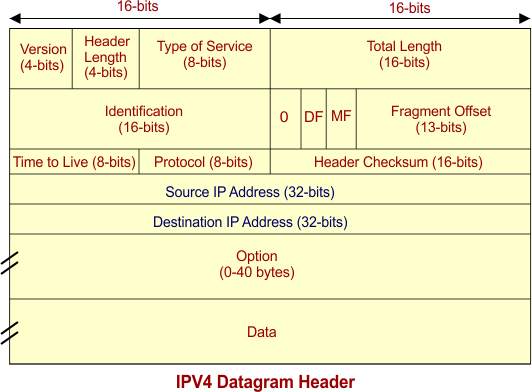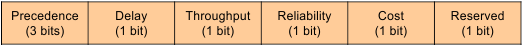Select Page

Datagram is a part of IPV4 Header. It represents the Header and payload size. Datagram of IPV4 is a 16 bit field which is equal to 65535 bytes. IPV4 Datagram is the combination of header size and payload  (data). IPV4 Datagram will always be in the range of 20 bytes to 65535 bytes (216 bytes).

The minimum size of header is 20 bytes or 160 bits and maximum of 60 bytes or 480 bits. First five rows of IPV4 header are mandatory, having total size of 20 bytes.

Each row is of 4-byte. So, first five rows size will be 20 bytes which is minimum size of IPV4 header.

The minimum size of Payload  (Data) is 0 bytes and maximum of 65515 bytes.

## IPv4 Datagram Header Size Calculations

• If header size is 20 bytes (minimum) and payload  is 0 bytes (minimum)  then datagram size = 20 bytes
• If header size is 20 bytes (minimum) and payload is 65515 (maximum) then datagram size = 65535 bytes
• If header size is 60bytes then the payload maximum value will be 65475. Because 65475 + 60 bytes are equal to maximum IPV4 datagram size which is equal to 65535.

Note: If header size is 60 bytes (maximum) and payload  is 65515 (maximum) then datagram size = 65575 bytes. it cannot represent by 16 bits field of datagram.

IPv4 Datagram Header diagram is given below1. VERSION: Version of the IP protocol is of 4 bits. These 4 bits are always fixed as 0100 to represent 4 in decimal for IPv4.

2. HLEN:IV4P header length is of 4 bits. The minimum value for this field is 5 and the maximum is 15 bytes.

• header length can be calculated by the following formula

Examples

If header length field contains decimal value 11 (represented in binary 1011) then Header length = 11 x 4 = 44 bytes

Note: So decimal range of 4-bits will becomes [5 -15] to represent header length [20-60]

3. Type Of Service: it is 8 bit field that is used for Quality of Service. The division of 8-bits are explained under• Precedence (3 bits): First 3 bits define the precedence. Precedence means priority i.e. immediate, routine etc. If a router is congested and needs to discard a packets, it will discard packets having lowest priority first. Bits values will be 0 or 1.
• Delay (1 bit): if we want a minimum delay in data packets then this field will be 1 otherwise 0. It Mostly in video calling where needs no delay.
• Throughput (1 bit): if need highly output then its field bit will be 1 otherwise 0.
• Reliability (1 bit): if need highly reliability then its field bit will be 1 otherwise 0. It is used where no data loss is tolerated.
• Cost (1 bit): if need low cost then its field bit will be 1 otherwise 0. It requires when to select the shortest path to its destination.
• Last bit is reserved for future purpose which mostly controls the congestion Notification. Congestion mean it inform to sender to minimize the speed of sending data.

4. Total Length: It is Total length of the datagram. it is a 16 bit field which can represent 216 = 65536 value . It has minimum size of 20 bytes and max value of 65535 bytes. As we already discuss in datagram topic that

5. Identification

• It is a 16-bit field. It is helpful for the identification of the fragments of an original datagram.
• When an IP datagram is fragmented, each fragmented datagram is assigned the same identification header number.
• This header-number is useful during the re-assembly of fragmented datagrams.

6. Flag Bits: It use 3 flag bits

• First flag bit is Reserved
• Second Flag bit (DF Bit). DF bit stands for Do Not Fragment bit. DF value may be 0 or 1.

When DF value is 0 then It gives the permission to the intermediate devices (i.e. routers) to fragment the datagram if required.

When DF value is 1 then It indicates the intermediate devices (i.e. router) not to fragment the datagram at any cost.

• Third flag bit is MF. MF bit stands for More Fragments bit. MF value may be 0 or 1.

When MF bit value is 0 then It tells to the receiver that the current datagram-fragment is the last fragment and no more segment will appear of same datagram.

When MF bit value is 1 then it tells more fragments are still to come after this fragment. MF bit is set to 1 for all the fragments except the last one.

7. Fragment Offset: Fragment Offset is a 13 bit field. It tells the position of a fragmented datagram in the original un-fragmented IP datagram.

Fragment offset for a given fragmented IP datagram = Number of data bytes ahead of it in the original un-fragmented IP datagram

Hence, The 1st fragmented datagram has a fragment offset of zero.

8. Time to live: It is 8-bit field which prevents the datagram to go to loop. If a datagram go to loop then congestion can happens which cause the problem. So, Time to live (TTL) avoids in such stations.

According to TTL, 8-bit can represent 256 nodes. Therefore, datagram is self-loop can goes to 256 nodes, when datagram goes to a node, it is decremented by 1 in values. As the value reaches 0, the datagram is terminated.

9. Protocol: it is an 8-bit number that defines what protocol is used inside the IP packet. TCP, UDP, ICMP or IGMP protocols can be filtered on, although they are most common. Protocol number of ICMP is 1 (in binary 00000001), IGMP is 2 (in binary 00000010), TCP is 6 and UDP is 17.

10. Header Checksum: it is a 16 bits field. It is used for checking errors in the datagram header. At receiving end, it is used to know that receiving data is corrupted or not because data can lose or corrupt while passing through the network.

13. Option: Due to options field, datagram-header-size can be of variable length (20 bytes to 60 bytes). Optional information include as source route etc.

Help Other’s By Sharing…